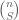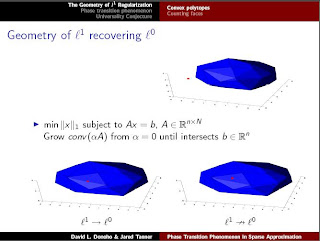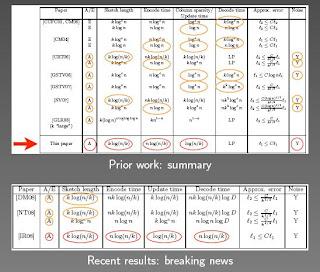## Page Views on Nuit Blanche since July 2010

My papers on ArXiv:
Approximating Kernels at the speed of Light
&
Imaging with Nature

LightOn
LinkedIn (727)|| on CrunchBase || our Blog
(2452)
(3967)
(1333)||
Attendant references pages:
The Advanced Matrix Factorization Jungle Page ||

Paris Machine Learning
@Meetup.com (8016 members) || @Archives

## Thursday, May 22, 2008

### CS: Restricted Isometry Property, What Is It Good For ?

Following the meeting at Texas A&M on Nonlinear Approximation Techniques Using L1, I have been trying to make sense of several presentations. If I have misinterpreted or misunderstood some aspect of the results presented, please let me know.

A matrix A is said to have the Restricted Isometry Property if for any k-sparse vector, the following expression is verified:The story goes that if A has this property, one can recover the sparse solution x of the underdetermined system A x = b using linear programming ( l_1) instead of a combinatorial search ( l_0). Hence, it is somewhat an important tool to guide engineers trying to implement compressed sensing on hardware. Take for instance the discussion in slide 16 to 22 of Roummel Marcia and Rebecca Willett's coded aperture architecture in Compressive Coded Aperture Superresolution Image Reconstruction (the slides are here).

Recently, Alexandre D'Aspremont pointed out that "... sparse PCA produces upper bounds on the restricted isometry constants ...", and therefore provided a potential elegant solution that showed that a specific matrix can follow the Restricted Isometry Property as opposed to the much less entertaining solution mentioned by Terry Tao a year ago:

An alternate approach, and one of interest on its own right, is to work on improving the time it takes to verify that a given matrix (possibly one of a special form) obeys the UUP. The brute-force approach of checking the singular values of every set of S column vectors requires a run time comparable to$\binom{n}{S}$ or worse, which is quite poor.

While convenient, this RIP property does not seem to be a very good tool to ascertain the usefulness of a matrix for Compressed Sensing (i.e. use "rapid" reconstruction techniques such as linear programming reconstruction codes). I have already mentioned something along these lines earlier ( CS: The KGG explanation of l0 and l1 correspondence without RIP, l_p minimization without a reference to RIP) but five presentations at the L1 conference seem to push the issue a little further.... I think.

Jared Tanner in Phase Transition Phenomenon in Sparse Approximation presented a geometrical approach to the correspondence between l_1 and l_0 with the folding of an l_1 ball into a polytope and then showed that Gaussian matrices were not the onlyfamily of measurement matrices that followed a certain empirical law for recovery: Partial Fourier as well as Sparse matrices  seem to yield similar bounds for the l_1 to l_0 recovery based on these geometric arguments.Using empirical evidence, he then shows that the Restricted Isometry Property is too strict of a property for a measurement matrix (red line in the graph below).In Enhanced Compressive Sensing and More, Yin Zhang asked the question of how one could deal with Compressed Sensing problems using additional prior information other than pure sparsity or compressibility,and developed a proof based on KGG mentioned earlier (CS: The KGG explanation of l0 and l1 correspondence without RIP, l_p minimization without a reference to RIP) in order to provide a more rapid way of proving the adequacy of Gaussian matrices for the recoverability of solutions when solving the l_p problem (for p less than 1) instead of the l_0 one. This is interesting as there are not that many results for these l_p metrics.He then showed how one can develops more advanced solvers in order to deal with these more complex situations [one of these solvers looks like ProxIT by the way]As an aside, I personally liked very much the use of prior information such as a similar signal to produce a quicker solution technique.Anna Gilbert, on the other hand, was featuring the much awaited paper that bridges the gap between the deterministic and non-deterministic approaches undertaken in Compressed Sensing ( the paper is " Combining Geometry And Combinatorics: A Unified Approach to Sparse Signal Recovery" and was written by Radu Berinde, Anna Gilbert, Piotr Indyk, Howard Karloff and Martin Strauss).

[ In the deterministic setting also known as Sketching, the sketch length corresponds to the number of rows of the measurement matrix. Also, one rarely builds the measurement matrix, it is implemented as an algorithm or a function call. Even if the matrix is actually sparse, it is still very very large. While the asymptotics look good, one of the reason you haven't seen much of the deterministic codes implementation in the big picture page (here or here) is because some of the constants involved in the asymptotics may be very bad. I am sure the deterministic folks can correct me on this paragraph, however as I have said in the past, if the engineers don't have one of these programs in their hands, one will never know if there is a niche market for them. hint hint]A summary of the deterministic methods and different bounds for CS is given in the following slide:In order to bridge the gap between the two approaches, the authors defined an RIP based on the l_1 norm and an RIP based on the l_2 norm.The fascinating part of this presentation was when it was shown that empirically sparse measurement matrices  obtained from the RIP(1)/deterministic setting seem to have similar behavior as the dense measurement matrices RIP(2) such as the Gaussian ensemble ! [ for illustration, see the exchange in the comment section of Raghunandan Kainkaryam's blog entry between Raghunandan and Radu Berinde] .These empirical results can be found in . Finally, the summary of the paper is very illuminating.In summary, RIP(1) is not the same as RIP(2), i.e. both properties do not yield the same ensemble of measurement matrices that allow signal reconstruction in CS using Linear Programming. Yet, one discovers that matrices that follow either RIP (1) or RIP(2) are very different in structure and yet seem to follow the same empirical performances. Jared Tanner shows a similar behavior and Yin Zhang bypasses entirely the need for RIP in order to attain results on the recoverability from l_p to l_0. In effect, it looks as though the Restricted Isometry Property is a very restrictive sufficient condition for recovering signals using l_1 instead of l_0. It is likely not a necessary condition. Hence, it may also be too restrictive to guide engineering work. For instance, I did not see it as a guiding principle in the Camera implementation proposed by Justin Romberg in his presentation on Architectures for Compressive Sampling. While the presentation of Gitta Kutyniok entitled "l1-Minimization and the Geometric Separation Problem"avoided the subject by estimating some type of partial coherence within the dictionaries.

Food for thoughts: Since the Restricted Isometry Property is more or less a statement on the eigenvalues of the measurement matrix (actually its 2-norm), what if the restrictiveness of this property could be alleviated by evaluating some of the properties of its eigenvectors without computing them ? For instance one could easily compute the pseudospectrum of these measurement matrices. The underlying thinking is as follows: The pseudospectrum is really a measure of how certain eigenvectors are nearly co-linear to each other. Graphically, the pseudospectrum represents the different level sets of the resolvent of a matrix/operator. If the pseudospectrum has level sets directly proportional to how far the contours are from every eigenvalues, then the operator is normal and all eigenvectors are orthogonal or nearly orthogonal to each other. If on the other hand, the level sets of the norm of the resolvent behave "abnormally" in the neighborhood of certain eigenvalues, then the group of eigenvectors related to these eigenvalues is nearly co-linear to each other. This colinearity property is something we want to avoid in dictionaries so I wonder if it would make sense to draw some conclusion between a statement on the pseudospectrum and the ability for measurement matrices to recover solutions using linear programming. Different methods have been devised to compute the pseudospectra of rectangular matrices  and that closer to home, Mark Embree at Rice has worked on this . Let us note the use of random matrices to compute pseudospectra. More information can be found at the Pseudospectra Gateway.

References:

 Sparse Recovery Using Sparse Random Matrices by Piotr Indyk and Radu Berinde.
Eigenvalues and Pseudospectra of Rectangular Matrices by Thomas G Wright and Lloyd N Trefethen
 Spectra and Pseudospectra: The Behavior of Nonnormal Matrices and Operators, Lloyd N Trefethen and Mark EmbreeAnonymous said...

Regarding the estimation of the restricted isometry constant, I do not understand how using sparse PCA can find a useful bound on RIP. Sparse PCA, if we could solve it, which we can't because it is probably NP hard, would evaluate a tight bound on the largest eigenvalue of submatrixes of a matrix. Call this bound s say.

We then have the bound (for x sparse)

(1-d)||x||_2^2
<=||Px||_2^2
<=s||x||_2^2
<=(1+d)||x||_2^2

Note that because the estimate of s is tight, i.e. by definition, there exist a sparse x, such that ||Px||_2^2=s||x||_2^2, the definition of RIP only guarantees that 1+d>=s, but this inequality does not have to be tight. (In the definition of RIP, either the lower or the upper bound is tight, but not necessarily both!) Therefore, s does not seem to give any useful bound on d.

Let me give a counter example, in the discussion, we have not placed any restrictions on the matrix P. In fact, it is easy to generate a matrix (call it D) for which s<=1. This clearly gives a useless bound s, which implies only that d>0. To generate D, take any matrix P and set D=P/||P||_2. With this normalisation, we have a matrix that satisfies

||Dx||_2^2<=1, for all x and therefore, also for all sparse x.

Furthermore, assume that P is such that for all sparse x, P has RIP constatn d, then for all sparse x,

(1-d)/||x||_2^2 ||x||_2^2 <= ||Dx||_2^2<=1

D has s=1, but RIP constant 1-((1-d)/||x||_2^2).Anonymous said...

In addition to my previous post, looking through Alexandre d'Aspremont's paper "Optimal Solutions for Sparse Principal Component Analysis", the problem in the previous post seems to stem from a possible error where on page 17 in arXiv:0707.0705v4, the authors state that, in the above notation, (1+d)<=s, instead of (1+d)>=s, as I think it should be. I could be wrong, however, I would need a strong argument to convince me that sparse PCA gives an upper and not a lower bound on (1+d).Anonymous said...

I agree that sparse factors produce lower bounds. However, the dual of the sparse PCA relaxation produces upper bounds. The strong argument you are looking for here is duality.
Alex

Igor said...

Anonymous,

Alex tells me offline that we will see more on the subject by the end of June.

Igor.

Piotr said...

Hi Igor,

Thanks for surveying Anna's talk. One comment: the paper, especially the notion of RIP1, provides a connection between *combinatorial approaches* (using sparse matrices and greedy recovery) and *geometric approaches* (using dense matrices and L1 minimization). The deterministic vs non-deterministic/randomized dichotomy is not as relevant here, since most of the combinatorial algorithms known in the sketching/streaming literature are actually randomized (e.g., CCFC'02, CM'04, CM'06 in the summary slide).

Still, it is true that most the of deterministic (or explicit) constructions of measurement matrices yield sparse matrices. One plausible explanation for this phenomenon is that one can build measurement matrices using expander graphs (which are sparse), and explicit construction of expander graphs is a major research direction in Theoretical Computer Science. If you only got a hammer, everything looks like a nail :)

Igor said...

Piotr,

Thanks for steering me in the right path. Yes it is an issue between geometric and combinatorial constructions and they so happen to be different because the TCS folks have tools dedicated to building sparse tools. I'll try to change the entry to reflect this. Finding an RIP(1) dense matrix or an RIP(2) sparse matrix would indeed be interesting.

Thanks again,

Igor.

Piotr said...

Finding an RIP(1) dense matrix or an RIP(2) sparse matrix would indeed be interesting.

Yes, it would. Personally though, I suspect that it might be impossible to get sparse AND efficient RIP(2) matrices. Specifically, I suspect that the results of [Chandar'08] can be extended to show that any sparse matrix satisfying RIP(2) must consist of many measurements (right now his proof holds only for 0-1 entries). If so, then one would not be able to get a matrix which is efficient, sparse and satisfying RIP(2).

Note that the construction of RIP(2) matrix by DeVore gives a sparse matrix, but the number of measurements in quadratic in the sparsity parameter.